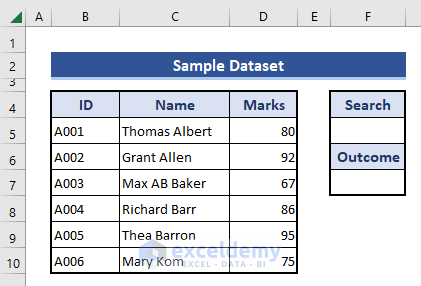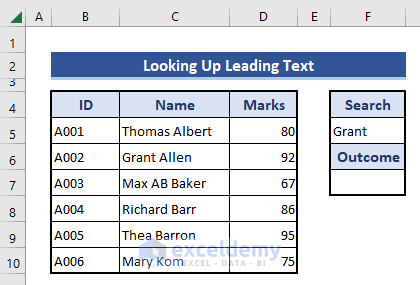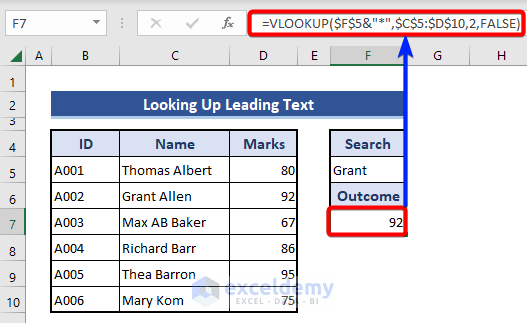# Wildcard with VLOOKUP in Excel (6 Examples)

Get FREE Advanced Excel Exercises with Solutions!

Excel is one of the most widely used software applications in our life. We use Excel to make data more meaningful and usable. In this article, we will discuss VLOOKUP with a wildcard. In Excel. VLOOKUP is commonly used to find data from big data sheets or multiple sheets with some clues.

To explain this topic, we used a data set of some students, their IDs, and their marks in an examination.

## Introduction to VLOOKUP

VLOOKUP looks up a value in the selected range of cells in the left-most column & returns the value in the same row in the index-number position.

• Syntax

=VLOOKUP(lookup_value,table_array,col_index_num,range_lookup)

• Argument

lookup_value – The value we want.

table_array – The table of data contains information from which we want the output. The table array should contain at least two columns of data. The first column contains the lookup values. These values can be text, numbers, or logical values.

col_index_num – It is the column from which we want value.

range_lookup – Range lookup is a logical value. It instructs VLOOKUP to find a match. The table must be sorted in ascending order. It offers two choices true or false. True for Approximate match and False for Exact match.

## Types of Wildcards

We have a total of 3 wildcard characters that are used in Excel.

Asterisk (*) – It searches any number of characters after a text.

Question Mark (?) – This question mark is used to replace a single character.

Tilde (~) – It can nullify the impact of the above two characters.

## 6 Examples of VLOOKUP with Wildcard in Excel

We will use the mentioned wildcards with VLOOKUP in Excel. The following examples will explain the use of those wildcards. For that, add new cells in the dataset to get the outputs.

##### Example 1: Looking for Leading Text based on Wildcard

In this example, we will look for a match based on the first word. But the VLOOKUP does not return without an exact match. For that, we will use a wildcard. That wildcard is the Asterisk (*). Look at the below steps for details.

📌 Steps:

• First, we insert the first word from any cell of the Name column on Cell F5.

##Here, we inserted the first word from Cell C6. The rest of the words are not inserted here.

• Now, look at the following formula used on Cell F7.

`=VLOOKUP(\$F\$5&"*",\$C\$5:\$D\$10,2,FALSE)`

##We selected Range C5:D10 for matching. Our reference of Cell F5 will find the match from the 1st column of the mentioned range. The third argument 2 is to get the return from the 2nd column. And the final argument is FALSE for getting the exact match. We already mentioned that we used only the 1st word for matching references. We used an asterisk symbol in the formula after Cell F5, which helps to apply the VLOOKUP formula.

### Example 2: Looking for Trailing Text in VLOOKUP based on Wildcard

Here, we will look for trailing text with the asterisk symbol. As we are inserting trailing text, we need to use the asterisk before the cell reference of the matching text in the formula.

📌 Steps:

• We insert the trailing text of Cell C10 on Cell F5 for reference.• Now, look at the formula of Cell F7.

`=VLOOKUP("*"&\$F\$5,\$C\$5:\$D\$10,2,FALSE)`In the formula, we inserted an asterisk symbol on the first of the lookup_value argument of the VLOOKUP function. The asterisk assumes the texts before the given reference based on the matching on the Name column.

### Example 3: Looking Up Middle Text Using Wildcard

In this example, we will look up using the middle test with the VLOOKUP function in Excel. This situation is quite different from the previous two examples. In the previous two examples, we used the wildcard 1 time in the formula. But here, we need to use wildcards two times. And the lookup values will locate between two wildcards in the formula.

📌 Steps:

• We insert the middle text of Cell C7 on Cell F5.• See the formula of Cell F7.

`=VLOOKUP("*"&\$F\$5&"*",\$C\$5:\$D\$10,2,FALSE)`We can see the asterisk is used two times in the formula. Also, the cell reference is located between the two asterisks. Due to two asterisks, the lookup_value will be set depending on the matching of the middle text. The asterisks will add more text before and after the given reference.

### Example 4: Add the Specific Number of Characters with Look Value

Previously, we used asterisks to add the remaining all text string with the look-up reference. But here, we need to add a certain number of characters using the wildcard. That wildcard is the Question mark (?). Look at the below section for details.

📌 Steps:

• We will look up based on the ID column. All the elements of the ID column are 4 digits.
• We input a 3-digit reference value on Cell F5 for lookup.
• We also set the format of the cell as Text.• Look at the formula of Cell F7.

`=VLOOKUP("?"&\$F\$5,\$B\$5:\$D\$10,3,FALSE)`This question mark will add characters with the lookup value after getting a match. The number of characters will depend on the number of question marks.

### Example 5: Avoid Trailing Space Using Wildcard in VLOOKUP

Sometimes it is seen that even after inserting the exact lookup value, the VLOOKUP function does not work. This may occur if there are unnecessary spaces in the lookup array. One more thing, if the spaces are at the end of cells, the spaces will not visible. To solve this problem, we can use the wildcard in the VLOOKUP formula.

📌 Steps:

• We input the look-up value at Cell F5, which is similar to Cell B5.We will also notice the trailing spaces of Cell B5 in the editable.

• Now, look at the formula of Cell F7.

`=VLOOKUP(\$F\$5,\$B\$5:\$D\$10,3,FALSE)`We get an error in the result despite using the exact text in the look-up section. This is because the trailing spaces are not without editable mode.

• Modify the applied formula and add an asterisk at the end of the lookup_value argument. The formula will look like this:

`=VLOOKUP(\$F\$5&"*",\$B\$5:\$D\$10,3,FALSE)`Now, we get the desired result.

### Example 6: Nullify the Effect of Previously Applied  Wildcards in Excel

In this example, we will show how Tilde(~) nullifies the effect of previously used other wildcards in Excel.

📌 Steps:

• We can see two cells in the Name column with asterisk wildcards.• Input Jon* on Cell F5.
• Then, insert the following VLOOKUP formula on Cell F7.

`=VLOOKUP(\$F\$5,\$C\$5:\$D\$10,2,FALSE)`But we get the return of Jony*. There is another close match with Cell C7. But due to the cell position, we get the return based on Cell C6.

• Now, we will use the Tilde(~)  to get the exact result nullifying the effect of the wildcard. Modify the lookup value of Cell F5.## How to Look Up Vertically with Wildcards and Return All Partial Matches in Excel

Sometimes it is seen that there are multiple matches in the look-up array. So, we need to show all the matches at that time. In this section, we will show how to look up vertically and return multiple partial matches in Excel. We will avoid the VLOOKUP function and use a customized formula. This formula is the combination of the IFERROR, INDEX, SMALL, IF, SEARCH, and ROW functions.

📌 Steps:

• We input 01 on Cell F5 for a partial match with the ID column.• Now, put the formula on Cell F7.

`=IFERROR(INDEX(\$D\$5:\$D\$10,SMALL(IF(IFERROR(SEARCH(\$F\$5,\$B\$5:\$B\$10)>0,FALSE),ROW(\$B\$5:\$B\$10)-ROW(\$B\$5)+1),ROW(1:1))),"")`This will return from Column C.

• Now, we will formula on Cell H5 after modifying the lookup array from Range C5:C10 to Range D5:D10.

`=IFERROR(INDEX(\$D\$5:\$D\$10,SMALL(IF(IFERROR(SEARCH(\$F\$5,\$B\$5:\$B\$10)>0,FALSE),ROW(\$B\$5:\$B\$10)-ROW(\$B\$5)+1),ROW(1:1))),"")`• Now, extend those inserted formulas through Columns G and H.

`=IFERROR(INDEX(\$C\$5:\$C\$10,SMALL(IF(IFERROR(SEARCH(\$F\$5,\$B\$5:\$B\$10)>0,FALSE),ROW(\$B\$5:\$B\$10)-ROW(\$B\$5)+1),ROW(3:3))),"")`After expanding the formulas we can see the last argument has been changed into 3:3.

Formula Explanation:

• ROW(1:1)

The ROW function returns the row number of the reference.

Result: 1

• ROW(\$B\$5)

This returns the row number of the given cell reference.

Result: 5

• ROW(\$B\$5:\$B\$10)

This returns an array of the given reference array.

Result: [5,6,7,8,9,10]

• SEARCH(\$F\$5,\$B\$5:\$B\$10)

The SEARCH function looks for the reference from the mentioned array and returns the number of occurrences.

Result: [3,#VALUE,3,#VALUE,3,#VALUE]

• IFERROR(SEARCH(\$F\$5,\$B\$5:\$B\$10)>0,FALSE)

Here, we compare the result of the SEARCH function with Zero (0). If the result of the SEARCH function is greater than zero, then shows TRUE. Otherwise, set that FALSE using the IFERROR function.

Result:[TRUE,FALSE,TRUE,FALSE,TRUE,FALSE]

• IF(IFERROR(SEARCH(\$F\$5,\$B\$5:\$B\$10)>0,FALSE),ROW(\$B\$5:\$B\$10)-ROW(\$B\$5)+1)

The IF and ROW functions are added to the formula. This shows the row number where this formula founds the match value.

Result: [1, FALSE, 3, FALSE, 5, FALSE]

• SMALL(IF(IFERROR(SEARCH(\$F\$5,\$B\$5:\$B\$10)>0,FALSE),ROW(\$B\$5:\$B\$10)-ROW(\$B\$5)+1),ROW(1:1))

The SMALL function returns the smallest value of the found matches.

Result: 1

• INDEX(\$C\$5:\$C\$10,SMALL(IF(IFERROR(SEARCH(\$F\$5,\$B\$5:\$B\$10)>0,FALSE),ROW(\$B\$5:\$B\$10)-ROW(\$B\$5)+1),ROW(1:1)))

The INDEX function returns the value from the lookup array based on the row number.

Result: Economics

• IFERROR(INDEX(\$C\$5:\$C\$10,SMALL(IF(IFERROR(SEARCH(\$F\$5,\$B\$5:\$B\$10)>0,FALSE),ROW(\$B\$5:\$B\$10)-ROW(\$B\$5)+1),ROW(1:1))),””)

The IFERROR function restricts from showing invalid values.

Result: Economics

## Things to Remember

• The Asterisk (*) can return any number of characters.
• The Question mark (?) returns characters based on the number of question marks.
• The Tilde(~) can nullify any or both of the wildcards.
• The VLOOKUP has two match types. One is approximate, and the other is the exact match. In all formulas used in this article, we choose exact matching for that we used the FALSE argument in the formula. If we use TRUE for approximate matching, the wildcard will not work properly.
• Another thing with the VLOOKUP function is- the lookup array will be the 1st column of the operation.

## Conclusion

In this article, we described how to use the wildcard with VLOOKUP in Excel. I hope this will satisfy your needs. Please have a look at our website ExcelDemy and give your suggestions in the comment box.

## Related Articles#### Alok Paul

Hello, this is Alok. I am working as an Excel & VBA Content Developer at Exceldemy. I want to provide solutions to various Excel-based problems. I completed my study at East West University major in Telecommunications Engineering. I love traveling, reading books, playing cricket.

We will be happy to hear your thoughtsAdvanced Excel Exercises with Solutions PDF